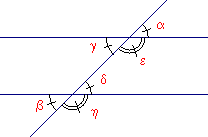www.batmath.it

A Glossary of Mathematical Terms - A

Abscissa

The first element in a coordinate pair; frequently called the x coordinate.

Absolute value

The absolute value of a number is the number itself if the number is non-negative, otherwise it is the opposite of the  number. It can also be described as the distance from zero. The absolute value is always non-negative.

Acute

See Angle or Triangle.

Two numbers whose sum is 0.

Two angles that have a common side in the interior of the angle formed by the two non common sides. (Pay attention: in Italian two angles are "adiacenti" if, in addition, they are supplementary; see Linear pair of angles).

3 plus 2 equals 5: 3+2=5;
3 increased by 2 is 5: 3+2=5;
2 more than 3 is 5: 3+2=5;
the sum of 3 and 2 is 5: 3+2=5;
the outcome in addition is called the sum;

See Angle.

Altitude (height)

of a triangle: the perpendicular segment from a vertex to the line containing the opposite side of the triangle
of a trapezoid: the distance between the two bases of the trapezoid;
of a cylindric solid: the distance between the planes of the bases;
of a conic solid or a pyramid; the distance between the vertex and the base.

AngleThe plane region between two rays that have the same endpoint; the two rays are called the sides of the angle;
it is measured in degrees or radians;
acute angle: lesser than 90°;
right angle: 90°;
obtuse angle: between 90° and 180°;
convex angle: lesser than 180°
straight angle: 180° (its two sides form a line);
reflex angle or concave angle: greater than 180°;
complementary angles: when the sum is 90°;
supplementary angles: when the sum is 180°;
alternate (alternating) interior angles: γ and δ;
alternate (alternating) exterior angles: α and β;
corresponding angles: α and δ; η and ε;
opposite (vertically opposite) angles: β and δ;
adjacent: two angles that have a common side in the interior of the angle formed by the two non common sides;
linear pair: two adjacent angles whose sum is 180°;

Array

An ordered arrangement of persons, things, rows and columns.

Angle bisector

A ray that is in the interior of angle and forms two equal angles with the sides of that angle.

Antecedent

In a conditional expression: the if part;
in a proportion: the first term of each ratio.

Arithmetic sequence (or progression)

A sequence of numbers where each term is the sum of the preceding number and a fixed value, called common difference.

Associative law (property)

The law states that when performing an operation on three or more objects, the result is unchanged by the way the objects are grouped. Addition and multiplication between numbers are associative, subtraction and division are not associative.

Average (mean)

There are various kinds of averages. The arithmetic average of n numbers is the sum of the numbers, divided by n. Usually average (or mean) is used in the sense of arithmetic average.

Axis (pl.axes)

The two lines that form the quadrants of the coordinate plane. Usually they are orthogonal and are, respectively, horizontal (x-axis) and vertical (y-axis).

first published on september 01 2003 - last updated on september 01 2003